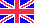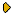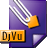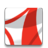Журнал Экспериментальной и Теоретической Физики
 НАЧАЛО | ПОИСК | ДЛЯ АВТОРОВ | ПОМОЩЬОбщая информация о журналеЗолотые страницыАдреса редакцииСодержание журналаСообщения редакцииПравила для авторовЗагрузить статьюПроверить статус статьиЖЭТФ, Том 136, Вып. 4, стр. 684 (Октябрь 2009)
(Английский перевод - JETP, Vol. 109, No 4, p. 585, October 2009 доступен on-line на www.springer.com )

RESONANCE REFLECTION BY THE ONE-DIMENSIONAL ROSEN-MORSE POTENTIAL WELL IN THE GROSS-PITAEVSKII PROBLEM

Поступила в редакцию: 13 Мая 2009

PACS: 05.30.Jp, 03.75.Lm, 03.75.Hh, 03.65.GeDJVU (68.8K)PDF (207.2K)

We consider the quantum above-barrier reflection of a particle by the one-dimensional Rosen-Morse potential well, for the nonlinear Schrödinger equation (the Gross-Pitaevskii equation) with a small nonlinearity. The most interesting case is realized in resonances when the reflection coefficient is exactly equal to zero for the linear Schrödinger equation. Then the reflection is determined by only a small nonlinear term in the Gross-Pitaevskii equation. The simple analytic expression is obtained for the reflection coefficient produced only by the nonlinearity. The analytic condition is found for the common action of the potential well and the nonlinearity to produce the zero reflection coefficient. The reflection coefficient is also derived analytically in the vicinity of a resonance shifted by the nonlinearity.

Сообщить о технических проблемах